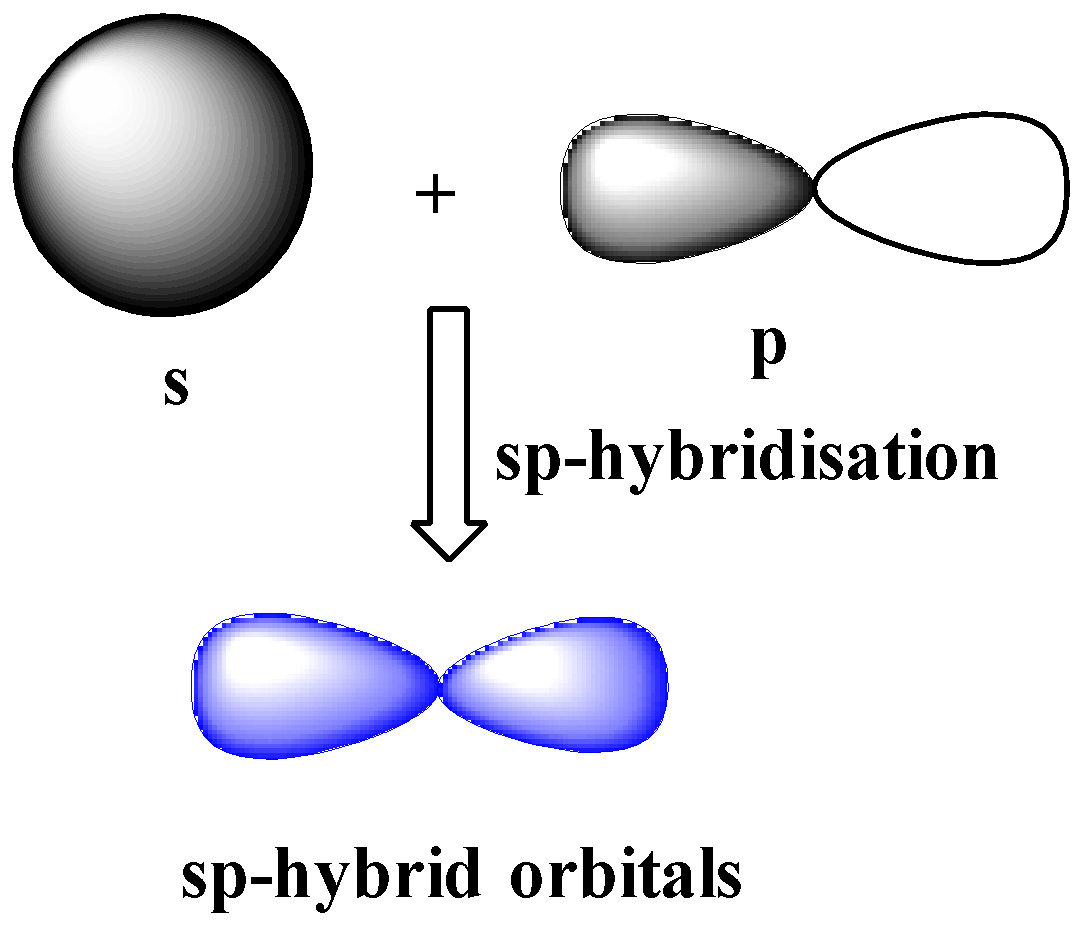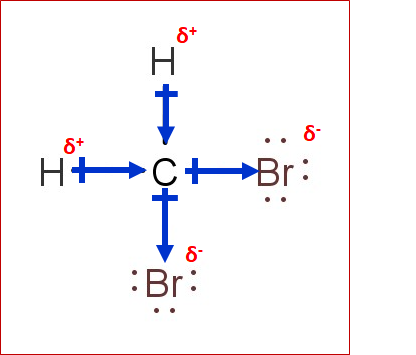# CH2Br2 Lewis Structure, Geometry, Hybridization, and PolarityCH2Br2 is a covalent compound formed by the bromination of methane. Dibromomethane is a colorless liquid with a pleasant odor. It is used to manufacture pharmaceutical compounds, fragrances, agrochemicals, etc.

In this article, we will study the basic concepts of prediction of Lewis structure, geometry, hybridization, and polarity.

Contents

## Lewis Structure

It is the 2D representation of a molecule. According to this concept, only valence shell electrons participate in bonding, and hence only they are represented while drawing the lewis structure of an atom. Lewis structures are one of the primitive attempts for the explanation of bonding.

Some compounds can have more than one Lewis structure. We need to satisfy the octet rule and formal charges for the most stable Lewis structure.

## Octet rule

All atoms form bonds to attain stability. Noble gases have eight valence electrons (except Helium), and they are considered stable.

Atoms lose or gain electrons to have eight electrons in their valence shell to attain a noble gas-like configuration.

This preference for eight electrons is the octet rule.

## Formal Charge

It is a theoretical concept. It compares the electrons present on an isolated neutral atom with the electrons present on the atom in the compound. The formula for the formal charge is

Formal charge = (total number of valence shell electrons in an isolated atom) – (number of nonbonded electrons)- 0.5*(number of electrons involved in bond formation)The formal charge is negative when the electrons on the atom in the compound are more than in an isolated atom and positive when the electrons on the atom in the compound are less than in an isolated atom.

When opposite charges are present on adjacent atoms, a bond can replace the charges.

A negative formal charge is more stable on an electronegative atom than on an electropositive atom and vice-versa.

## Steps to Draw Lewis Structure of CH2Br2

Step 1: First of all, count the number of electrons in the valence shell of each atom.

Before drawing the Lewis structure, we need to know the number of valence shell electrons on all constituent atoms and their sum.

Atom Atomic Number Group Number Valence electrons according to group number Electronic configuration (E.C.) Valence shell from E.C. Valence electrons from E.C.
One C 6 14 4 1s2 2s2 2p2 n=2 4
Two H 1 1 1 1s1 n=1 1
Two Br 35 17 7 1s2 2s2 2p6 3s2 3p6 3d10 4s2 4p5 n=4 7
Total number of valence shell electrons= 4 + (1*2)+(7*2) = 20

Step 2: Draw the lewis dot structure for elements.

We draw the Lewis structure of elements by arranging the valence shell electrons around the element’s chemical symbol.

The chemical symbols for carbon, hydrogen, and bromine are C, H, and Br, respectively. The Lewis dot structure for C, H, and Br are as follows-Step 3: Choose a suitable central atom for the compound.

We have to choose the central atom carefully. It must be the least electronegative atom out of the constituent atoms (C, H, Br).

If it is more electronegative than side atoms, the central atom will keep the electron density towards itself.

Bromine is the most electronegative out of constituent atoms and cannot be the central atom. Hydrogen can never be the central atom because it forms one bond only. Thus, C is the central atom for this compound.

Step 4: Draw a skeletal diagram.

In this step, we have to arrange the side atoms and central atoms suitably.Step 5: Arrange the valence electrons around the elemental symbols.

The total valence shell electrons (calculated in step 1) are placed according to a predicted bond formation.Step 6: Form bonds between adjacent atoms and try to complete the octet for all atoms.C has four valence electrons in the isolated state. It shares one electron with each H and Br to have a fully filled valence shell configuration.

Each H has one valence electron, and they share one with C to have a fully filled configuration.

Each bromine atom has 7 electrons in the valence shell in a free state, and it shares one electron with carbon to have a fully filled configuration.

All the atoms have a complete octet in dibromomethane.

Step 7: Calculate the formal charge on all atoms.

The net charge on this compound is zero.

Therefore, formal charge on C+ H1+ H2+ Br1+ Br2 = 0

 Atom Number of electrons in the valence shell according to the periodic table electrons in the valence shell according to the periodic table Number of electrons as lone pair (Number of electrons involved in bonding )*0.5 Formal Charge on atom C 4 0 8*0.5=4 4-0-4=0 H1 1 0 2*0.5=1 1-0-1=0 H2 1 0 2*0.5=1 1-0-1=0 Br1 7 6 2*0.5=1 7-6-1=0 Br2 7 6 2*0.5=1 7-6-1=0

Thus, the structure drawn in step 6 is the best Lewis structure for CH2Br2.

## CH2Br2 Geometry

Molecular geometry refers to the 3D arrangement of atoms of a molecule in space. Lewis structure does not predict the geometry of a compound, and hence we needed another theory. VSEPR theory helps in determining the shape and geometry of a given compound.

VSEPR theory stands for valence shell electron pair repulsion theory.

This theory is based on the principle that the valence shell electrons of each atom in a molecule arrange themselves in such a way that the inter-electronic repulsion is minimum, and the arrangement becomes stable. The stable arrangement is called geometry.

Geometry and shape are often confused with each other. Geometry refers to the arrangement of bond pair of electrons, while shape refers to the arrangement of bond pair and lone pair of electrons.

### The Geometry of CH2Br2 Using VSEPR

1. First of all, we have to count the electrons in the valence shell of the central atom and let them be equal to A (arbitrary variable)

In the case of CH2Br2, the central atom is C. C has 4 valence electrons. (Shown in step1 of drawing lewis structure)

A=4

2. Count the number of side atoms and let it be equal to B (arbitrary variable). In CH2Br2, there are four side atoms (two H and two bromine) and B=4

3. If the compound is charged, subtract the charge from B for the positively charged compound and add the charge to B for the negatively charged compound. This step can be avoided for neutral compounds.

In CH2Br2,  there is no contribution of charge and B=4 only.

4. Add the contribution of side atoms and charge to the contribution of the central atom, i.e., A+B

For CH2Br2, A+B = 8

5. Divide A+B by 2 to find total electron pairs affecting the shape.

For CH2Br2, there are 4 electron pairs.

6. Divide the total electron pairs as bonding and non-bonding. The bonding electron pair is equal to the number of side atoms.

For CH2Br2, there are four side atoms. Thus, there are four bonding pairs of electrons and zero nonbonding pairs of electrons.

Using this information, one can easily find the geometry and shape for dibromo methane from the table below.

Electron geometry and shape are tetrahedral. The geometry and shape are the same for compounds with zero lone pairs.## CH2Br2 Hybridization

Hybridization is one of the fundamental concepts used to explain the bond formation and was introduced by Pauling.

Hybridization involves the mixing of two or more atomic orbitals to form orbitals with the same energy, shape, and size. The hybridized orbitals form bonds, and the extent of overlap is better than unhybridized orbitals.

Only those orbitals can undergo hybridization which has similar energy, shape, and size.

For example, one 2s and three 2p can form four sp3 hybrid orbitals with the same size, shape, and energy, but 2s and 6d cannot.It does not involve literal mixing. Just mixing of wavefunctions takes place.

In CH2Br2, C is the central atom. We are focusing on the hybridization of the central atom only. In the ground state, C has 2 unpaired electrons. It can only form two bonds.

Promotion of electrons takes place, and all 4 valence electrons become unpaired.

These 4 electrons are present in different orbitals. All 4 orbitals undergo hybridization to form sp3 hybrid orbitals.### Steric Number Method

In this method, we have to find the total number of electron pairs on the central atom, as we did in the VSEPR method for determining geometry.

For dibromo methane, electron pairs=4

We also have a direct formula for steric numbers.

Steric number = number of (sigma bonds +lone pair on central atom)

Steric number for CH2Br2= (4+0)=4

Hybridization of CH2Br2 comes out to be sp3 from the table.Related Topics

H2O2 Lewis Structure

NO3 Lewis Structure

NO2 Lewis Structure

N2O Lewis Structure

H2SO4 Lewis Structure

CH3OCH3 Lewis Structure

PF3 Lewis Structure

NH3 Lewis Structure

N2 Lewis Structure

Br2 Lewis Structure

## CH2Br2 Polarity

We can say that a compound is polar if the net dipole moment is non-zero. Dipole moment is a vector quantity.

It depends on-

Dipole moment of individual bonds

• The difference in electronegativity of atoms constituting the bonds

• Geometry/ symmetry of compound

In CH2Br2, there are two types of bonds, ‘ C-H’ and ‘C-Br.’

According to the electronegativity scale of Linus Pauling-

• Electronegativity of C= 2.55

• Electronegativity of H=2.2

• Electronegativity of Br=2.96

The difference for ‘C-H’ and ‘C-Br’ comes out to be 0.35 and 0.41, respectively. Hence, we can say that the bonds are polar and the bond dipole moment is non-zero.

Polar bonds do not guarantee a polar molecule. It is a tetrahedral compound. The vector’s sum of the dipole moment of the four bonds comes out to be non-zero due to different substituents.

Hence, CH2Br2 is a polar molecule.## Conclusion

Dibromomethane is a useful organic compound.

The Lewis structure drawn in the above section is the most appropriate because it satisfies the octet rule and formal charges.

The geometry and shape come out to be tetrahedral for dibromomethane (CH2Br2).

Hybridization for the central atom in CH2Br2 is sp3.

It is a polar compound.